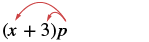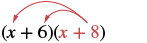## Multiplying Two Binomials Using the Distributive Property

### Learning Outcomes

• Use the distributive property to multiply two binomials

Just like there are different ways to represent multiplication of numbers, there are several methods that can be used to multiply a binomial times a binomial.

### Using the Distributive Property

We will start by using the Distributive Property. Look again at the following example.We distributed the $p$ to get $x\color{red}{p}+3\color{red}{p}$ What if we have $\left(x+7\right)$ instead of $p$ ? Think of the $x+7$ as the $\color{red}{p}$ above.Distribute $\left(x+7\right)$ .Distribute again. ${x}^{2}+7x+3x+21$ Combine like terms. ${x}^{2}+10x+21$

Notice that before combining like terms, we had four terms. We multiplied the two terms of the first binomial by the two terms of the second binomial—four multiplications.

Be careful to distinguish between a sum and a product.

$\begin{array}{cccc}\hfill \mathbf{\text{Sum}}\hfill & & & \hfill \mathbf{\text{Product}}\hfill \\ \hfill x+x\hfill & & & \hfill x\cdot x\hfill \\ \hfill 2x\hfill & & & \hfill {x}^{2}\hfill \\ \hfill \text{combine like terms}\hfill & & & \hfill \text{add exponents of like bases}\hfill \end{array}$

### example

Multiply: $\left(x+6\right)\left(x+8\right)$

Solution

 $\left(x+6\right)\left(x+8\right)$Distribute $\left(x+8\right)$ . $x\color{red}{(x+8)}+6\color{red}{(x+8)}$ Distribute again. ${x}^{2}+8x+6x+48$ Simplify. ${x}^{2}+14x+48$

### try it

Now we’ll see how to multiply binomials where the variable has a coefficient.

### example

Multiply: $\left(2x+9\right)\left(3x+4\right)$

### try it

In the previous examples, the binomials were sums. When there are differences, we pay special attention to make sure the signs of the product are correct.

### example

Multiply: $\left(4y+3\right)\left(6y - 5\right)$

### try it

Up to this point, the product of two binomials has been a trinomial. This is not always the case.

### example

Multiply: $\left(x+2\right)\left(x-y\right)$

### try it

To see another example of how to visualize multiplying two binomials, watch the following video. We use an area model as well as repeated distribution to multiply two binomials.